NEET  >  Test: Electrolysis & Faraday's Laws

# Test: Electrolysis & Faraday's Laws - NEET

Test Description

## 32 Questions MCQ Test Chemistry Class 12 - Test: Electrolysis & Faraday's Laws

Test: Electrolysis & Faraday's Laws for NEET 2023 is part of Chemistry Class 12 preparation. The Test: Electrolysis & Faraday's Laws questions and answers have been prepared according to the NEET exam syllabus.The Test: Electrolysis & Faraday's Laws MCQs are made for NEET 2023 Exam. Find important definitions, questions, notes, meanings, examples, exercises, MCQs and online tests for Test: Electrolysis & Faraday's Laws below.
Solutions of Test: Electrolysis & Faraday's Laws questions in English are available as part of our Chemistry Class 12 for NEET & Test: Electrolysis & Faraday's Laws solutions in Hindi for Chemistry Class 12 course. Download more important topics, notes, lectures and mock test series for NEET Exam by signing up for free. Attempt Test: Electrolysis & Faraday's Laws | 32 questions in 45 minutes | Mock test for NEET preparation | Free important questions MCQ to study Chemistry Class 12 for NEET Exam | Download free PDF with solutions
 1 Crore+ students have signed up on EduRev. Have you?
Test: Electrolysis & Faraday's Laws - Question 1

### Only One Option Correct Type This section contains 16 multiple choice questions. Each question has four choices (a), (b), (c) and (d), out of which ONLY ONE is correct Q. During the electrolysis of aqueous Zn(NO3)2 solution

Detailed Solution for Test: Electrolysis & Faraday's Laws - Question 1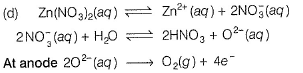In preference to reduction of Zn2+ at cathode, O2- (aq) is oxidised to O2 at anode.

Test: Electrolysis & Faraday's Laws - Question 2

### Schematic diagram of an electrolytic cell is

Detailed Solution for Test: Electrolysis & Faraday's Laws - Question 2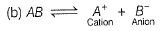In electrolytic cell,
Cation moves to cathode and anion moves to anode.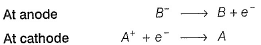Electrons move anode to cathode.

Test: Electrolysis & Faraday's Laws - Question 3

### The metal that can not be obtained by electrolysis of an aqueous solution of its salt is [JEE Main 2014]

Detailed Solution for Test: Electrolysis & Faraday's Laws - Question 3

In electro chemical series (ECS),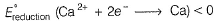thus, Ca2+ ion is not reduced to C a instead, H2O is reduce d to H2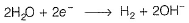Ag, Cu and Cr are below H in ECS (E°reduction > 0), thus they are reduced to metals on electrolysis.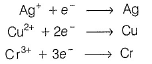Note In ECS, Ca, H, Cr, Cu elements lying above H are better reducing agents thus are easily oxidised.

Test: Electrolysis & Faraday's Laws - Question 4

When during electrolysis of a solution of AgNO3 ,9650 C of charge pass through the electroplating bath,the mass of silver deposited on the cathode will be

Detailed Solution for Test: Electrolysis & Faraday's Laws - Question 4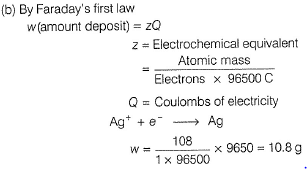Test: Electrolysis & Faraday's Laws - Question 5

Refining of impure metal is done by electrolysis using impure metal as anode.Select the correct statement about this refining.

Detailed Solution for Test: Electrolysis & Faraday's Laws - Question 5

When impure metal (M) is anode and pure metal is made cathode, then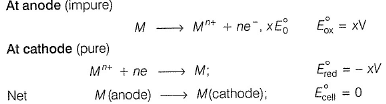Thus, impure metal from the anode is deposited at the cathode.

Test: Electrolysis & Faraday's Laws - Question 6

W g of Ag is deposited at the cathode of one electrolytic cell due to passage of 1A of current for 1h.Time required for passage of current to deposit W g of Mg by the same value of current is

Detailed Solution for Test: Electrolysis & Faraday's Laws - Question 6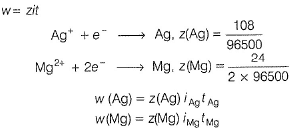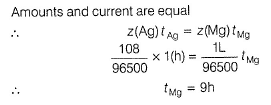Test: Electrolysis & Faraday's Laws - Question 7

In the refining of silver by electrolytic method, current of 5A is passed for 2 h using 100 g of impure anode of silver (of 95% purity). Weight of silver anode after electrolysis is

Detailed Solution for Test: Electrolysis & Faraday's Laws - Question 7

By Faraday 's first law of electrolysis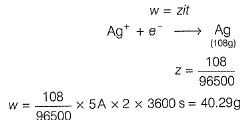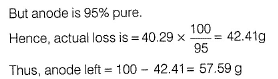Test: Electrolysis & Faraday's Laws - Question 8

In the electrorefining of metals, impure metal is

Detailed Solution for Test: Electrolysis & Faraday's Laws - Question 8

Impure metal is made the anode and oxidation takes place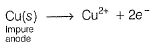Cu2+ formed are reduced at cathode and thus, deposited there.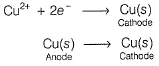Test: Electrolysis & Faraday's Laws - Question 9

In the electrosynthesis,potassium manganate (VII) is converted to manganese(IV) dioxide. By passage of 1F of electrolysis ,one mole of potassium manganate(VII) will form manganese dioxide.

Detailed Solution for Test: Electrolysis & Faraday's Laws - Question 9

Manganate (VII) is MnO4- with ON of Mn = + 7
1 mole required 3F of electricity thus, 1 F will reduce only (1/3) mole MnO4- t o MnO2.
Thus, MnO2 formed = 0.33 mol.

Test: Electrolysis & Faraday's Laws - Question 10

When Al2O3 is electrolysed ,cation and anions are discharged. For a given quantity of electricity,ratio of number of moles of Al and O2 gas is

Detailed Solution for Test: Electrolysis & Faraday's Laws - Question 10

When same quantity of electricity is passed, elements/gases are formed in the ratio of their equivalents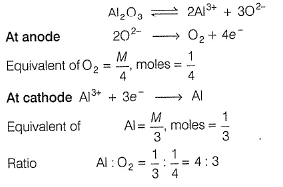.

Test: Electrolysis & Faraday's Laws - Question 11

1 Faraday of electricity is passed through the solution containing 1 mole each of AgNO3, CuSO4, AlCland SiCl4. Elements are discharged at the cathode.Number of moles of Ag, Cu,Al and Si formed will be in the ratio of

Detailed Solution for Test: Electrolysis & Faraday's Laws - Question 11

(d)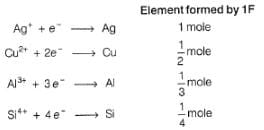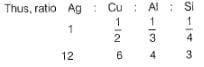Test: Electrolysis & Faraday's Laws - Question 12

During electrolysis of acidified water ,O2 gas is formed at the anode. To produce O2 gas at the anode at the rate of 0.224 cc per second at STP,current passed is

Detailed Solution for Test: Electrolysis & Faraday's Laws - Question 12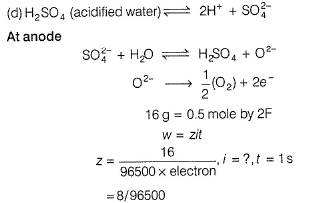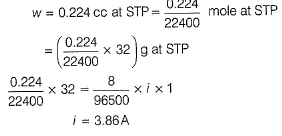Test: Electrolysis & Faraday's Laws - Question 13

A solution of copper(II) sulphate (VI) is electrolysed between copper electrodes by a currrent of 10.0 A for exactly 9650 s.Which remains unchanged?

Detailed Solution for Test: Electrolysis & Faraday's Laws - Question 13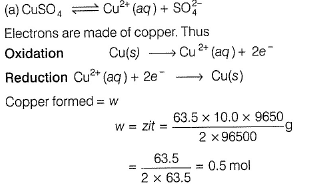0.5 mole of copper is dissolved from the anode. Thus, its mass decreases. 0.5 mole of copper from the anode is deposited at the cathode. Thus, its mass increases.
Thus, molar concentration of aqueous solution of CuSO4 remains unchanged.

Test: Electrolysis & Faraday's Laws - Question 14

A 300 mL solution of NaCl was electrolysed for 60.0 min. If the pH of the final solution was 12.24,average current used is

Detailed Solution for Test: Electrolysis & Faraday's Laws - Question 14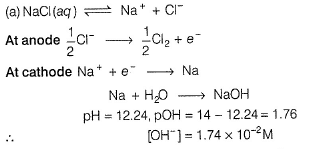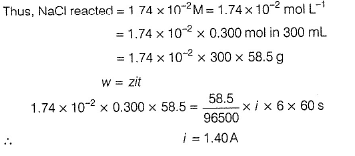Test: Electrolysis & Faraday's Laws - Question 15

100 mL of a buffer of 1 M NH3(aq) and 1 M NH4+(aq) are placed in two volatic cells separately.A current of 1.5 A is passed through both cells for 20 min. If electrolysis of water only takes place

2H2O +O2 + 4e- → 4OH- (RHS

2H2O  → 4H+. + O2 + 4e- (LHS)

then pH of the

Detailed Solution for Test: Electrolysis & Faraday's Laws - Question 15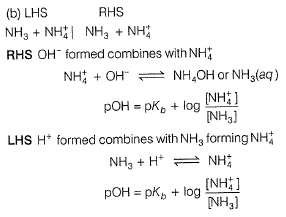In RHS, [NH3] increases hence, pOH decreases
pH = 14 - pOH
Hence, pH of RHS increases.
In LHS, [NH4+] increases, hence pOH increases and pH decreases.

Test: Electrolysis & Faraday's Laws - Question 16

What product are formed during the electrolysis of a concentrated aqueous solution of sodium chloride using an electrolytic cell in which electrodes are separated by a porous pot?

I. Cl2(g)
II. NaOH(aq)
III. H2(g)
IV. NaClO(aq)
V. NaClO3(aq)

Select the correct choice.

Detailed Solution for Test: Electrolysis & Faraday's Laws - Question 16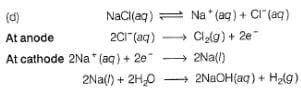Cl2(g) is formed at anode and NaOH and H2 are formed at cathode. Since, cathode and anode are separated. Hence. there is no reaction between the products formed at the cathode and anode.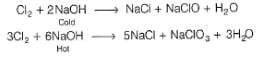Note: but these reaction do not occur

Note: NaCl is neutral pH = 7 initially. After electrolysis , NaOH is formed

Hence, solution become, basic pH >7

*Multiple options can be correct
Test: Electrolysis & Faraday's Laws - Question 17

One or More than One Options Correct Type

This section contains 5 multiple type questions. Each question has 4 choices (a), (b), (c) and (d), out of which ONE or MORE THAN ONE are correct.

Select the correct point(s) of distinction between a volatic cell and electrolysis cell.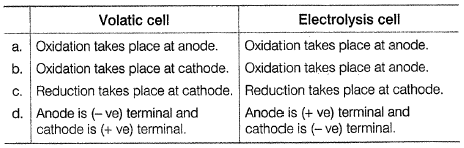Detailed Solution for Test: Electrolysis & Faraday's Laws - Question 17

(a.c.d) Regardlessvehether a cell is a volatic or an electrolysis cell, anode s the electrode at which oxidation takes place and cathode is the electrode at which reduction takes place. In electrochemical cell, Zn → Zn2+ + 2e- .

It is losing electrons thus, anode is negative terminal. In electrolysis cell. cation migrates to cathode thus -ye terminal. anion migrates to anode thus +ve terminal.

*Multiple options can be correct
Test: Electrolysis & Faraday's Laws - Question 18

In the electrolysis of aqueous sodium chloride solution, two types of reactions can take place at anode :

I. 2Cl- (aq) → Cl2(g) +2e-

II. 2H2O(l)g → O2 (g) + 4H+(aq) + 4e-

Select the correct statement(s) about these.

Detailed Solution for Test: Electrolysis & Faraday's Laws - Question 18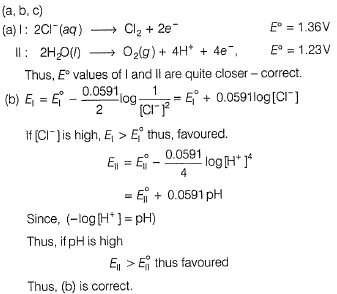(c) Experrnent indicates that the applied voltage required is always greater than the voltage calculated from the standard E0ox or E0red. The additional voltage required is called overvoltage. Due to this, Cl2 and not O2 s formed. This, (c) 6 correct.

*Multiple options can be correct
Test: Electrolysis & Faraday's Laws - Question 19

Select the correct statement(s) about electrolysis of aqueous CuSO4 solution.

Detailed Solution for Test: Electrolysis & Faraday's Laws - Question 19

(a)

Oxidation takes place at anode - correct

2H2O(l) →4H+ (aq) + O2(g) + 4e-

(b) Reduction take place at cathode - correct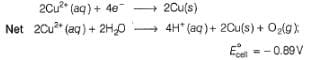since, E0cell < 0, in electrolysis.

Cell reaction is spontaneous.

(c) Copper is deposited at the cathode, hence the meted to be electroplated (say Fe), should be made cathode - correct (d) Since. Cu2+(aq)- (blue) is reduced Cu(s), hence blue colour fades - correct.

*Multiple options can be correct
Test: Electrolysis & Faraday's Laws - Question 20

Select the correct observation about electrolysis.

Detailed Solution for Test: Electrolysis & Faraday's Laws - Question 20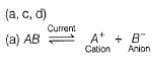Thus, electric current is used to carry out a non-spontaneous reaction.
Thus. (a) is correct.
(b) Since reactions are reverse of electrochemical cell, hence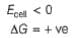thus, (b) is incorrect

(c) cation goes to cathode and anion goes to anode and are discharged. Thus, correct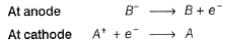(d) some additional voltage called overvoltage is set up and thus other reactions also take place. thus correct

*Multiple options can be correct
Test: Electrolysis & Faraday's Laws - Question 21

Which pair of electrolysis could be distinguished by the products of electrolysis using inert electrodes?

Detailed Solution for Test: Electrolysis & Faraday's Laws - Question 21

a(Cu So4 and CuCl2 changes to Cu and solution becomes colourless

(b) KCl changes to Cl2 (yellow)
KI changes to I2 (voilet)

Thus, distinguished.

(c) AgNo3 No change

CuSo4 (blue) changes to colourless

(d) CuBr2 (blue) both changes to colourles.

NiBr2 (Blue)

Test: Electrolysis & Faraday's Laws - Question 22

Comprehension Type

This section contains a passage describing theory, experiments, data, etc. Two questions related to the paragraph have been given. Each question has only one correct answer out of the given 4 options (a), (b), (c) and (d)

Passage I

A constant current of 30 A is passed through an aqueous solution of NaCl for a time of 1.00 h.

Thus NaOH formed due to electrolysis is

Detailed Solution for Test: Electrolysis & Faraday's Laws - Question 22

The correct answer is option B
Formula used : W=ZIt
where Z is the equivalent mass of the deposit divided by 1 Faraday charge i.e. 96500 C.

Now, W= [(40/{+1})/96500] x 30 x  3600s

≈ 44.8 g

Test: Electrolysis & Faraday's Laws - Question 23

Passage I

A constant current of 30 A is passed through an aqueous solution of NaCl for a time of 1.00 h.

Thus Cl2 formed under STP condition is

Detailed Solution for Test: Electrolysis & Faraday's Laws - Question 23

At anode 2Cl- —-±>Cl2+2e-
At cathode 2Na+ +2e- ——-> 2Na.
So 2 Faraday of electricity is required to liberate 1 mole = 22.4 liters of Cl2 at STP.
Amount of current passed
= 30 x 60 x 60=108000 coulombs.
So 108000 coulombs will liberate =108000/(96500 x2) = 0.5596 moles Cl2.
No.of liters of Chlorine liberated at STP
= 0.5596 x 22.4 = 12.53 liters.

Test: Electrolysis & Faraday's Laws - Question 24

Passage II

In hydrogen economy fuel-cell,anodic and cathodic reactions are

Anodic

H2+2OH- → 2H2O + 2e-

Cathodic

O2+2H2O + 2e- → 4OH-

67.2 L H2 at STP react in 15 min.Entire current is used for electro deposition of copper from copper(II) sulphate solution. Average current produced in fuel cell is

Detailed Solution for Test: Electrolysis & Faraday's Laws - Question 24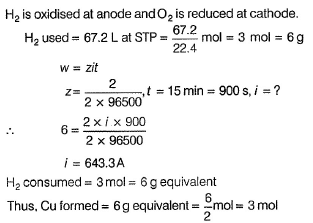Test: Electrolysis & Faraday's Laws - Question 25

Passage II

In hydrogen economy fuel-cell,anodic and cathodic reactions are

Anodic

H2+2OH- → 2H2O + 2e-

Cathodic

O2+2H2O + 2e- → 4OH-

67.2 L H2 at STP react in 15 min.Entire current is used for electro deposition of copper from copper(II) sulphate solution. Copper deposited would be

Detailed Solution for Test: Electrolysis & Faraday's Laws - Question 25*Answer can only contain numeric values
Test: Electrolysis & Faraday's Laws - Question 26

One Integer Value Correct Type

This section contains 6 questions, when worked out will result in an integer value from 0 to 9 (both inclusive)

Mn+ solution was electrolysed for 75 min by a current of 0.30 A. Mass of the metal M deposited at the cathode was 9.958 g.Mn+ contains 68 protons and 69 neutrons. What is the value of n?

Detailed Solution for Test: Electrolysis & Faraday's Laws - Question 26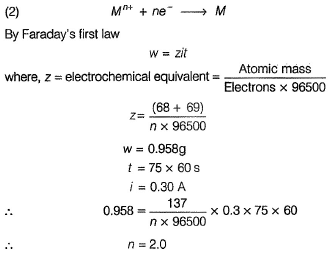*Answer can only contain numeric values
Test: Electrolysis & Faraday's Laws - Question 27

An electrochemical cell was based on the following reaction:

Mn(OH)2(s) + H2O2(aq) → MnO2(s) + 2H2O (e)

During the opeartion of this for 1 min, 0.135 g of MnO2 was produced. What is the average electric current (in ampere ) produced by the cell?

Detailed Solution for Test: Electrolysis & Faraday's Laws - Question 27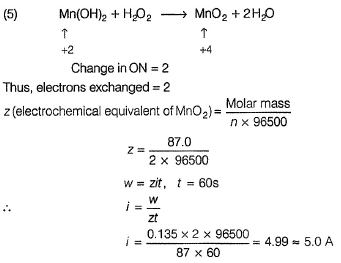*Answer can only contain numeric values
Test: Electrolysis & Faraday's Laws - Question 28

In a fuel cell, following reactions takes place and electricity is produced.

Anodic

H2+2OH- → 2H2O + 2e-

Cathodic

O2+2H2O + 4e- → 4OH-

If 100.8 L of H2 at STP reacts in 96500 s,what is the average current produced (in ampere)?

Detailed Solution for Test: Electrolysis & Faraday's Laws - Question 28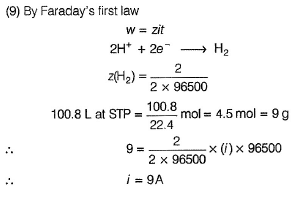*Answer can only contain numeric values
Test: Electrolysis & Faraday's Laws - Question 29

How long (in minutes ) a current of 1.56 A has to be passed through a solution of AgNO3 to coat a metal surface of 80 cm2 with a 0.010 mm thick layer? Density of silver is 10.5 g cm-3.

Detailed Solution for Test: Electrolysis & Faraday's Laws - Question 29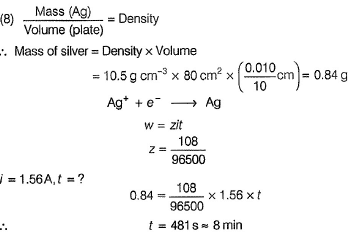*Answer can only contain numeric values
Test: Electrolysis & Faraday's Laws - Question 30

A fully charged battery contains 500 mL of 5.00 M H2SO4. What is the concentration of H2SO4 in the battery after 6.0 A of current is drawn from the battery for 13.40 h?

Detailed Solution for Test: Electrolysis & Faraday's Laws - Question 30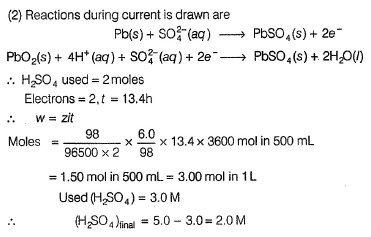*Answer can only contain numeric values
Test: Electrolysis & Faraday's Laws - Question 31

A hydrogen-oxygen fuel cell opeartes on the simple reaction: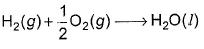If the cell is designed to produce 1.46A of current and if the H2 is contained in a 1.0 L tank at 2.00 atm pressure at 298K, how long can the fuel cell operate(in hour) before the H2 runs out.(Assume unlimited supply of oxygen)

Detailed Solution for Test: Electrolysis & Faraday's Laws - Question 31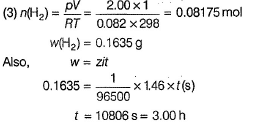Test: Electrolysis & Faraday's Laws - Question 32

Matching List Type

Choices for the correct combination of elements from Column I and Column II are given as options (a), (b), (c) and (d), out of which one is correct

Four electrolytic cells containing solution of ZnSO4, AgNO3, CuSO4 and dil. H2SO4 are connected in series. A steady current of 1.5 A was passed through them until 77 mL of O2 gas at STP is formed in anode compartment of H2SO4 solution. Match the different elements/gas formed In Column I with their values in Column II and select the answer from the codes given below. (Cu = 63.5, Zn = 65, Ag = 108, S = 32, H = 1, 0 = 16)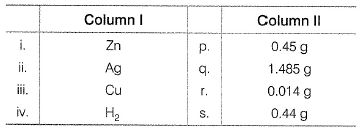Codes   i       ii      iii      iv

Detailed Solution for Test: Electrolysis & Faraday's Laws - Question 32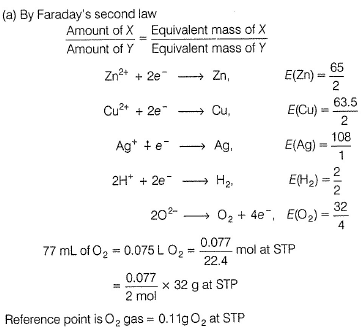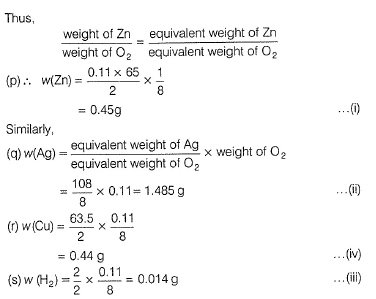## Chemistry Class 12

150 videos|377 docs|213 tests